# Regular n-gon

In a regular n-angle polygon, the internal angle is 144 degrees. Find the number n indicating the number of sides of this polygon.

n =  10

### Step-by-step explanation: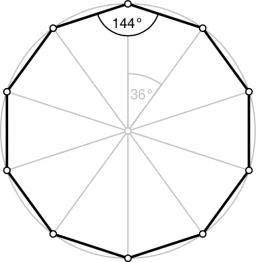Did you find an error or inaccuracy? Feel free to write us. Thank you!#### You need to know the following knowledge to solve this word math problem:

We encourage you to watch this tutorial video on this math problem:

## Related math problems and questions:

• Squares above sidesIn a right triangle, the areas of the squares above its sides are 169; 25 and 144. The length of its longer leg is:Calculate the content of a regular 15-sides polygon inscribed in a circle with radius r = 4. Express the result to two decimal places.
• 144 stepsTo get around the garden we have to take 144 steps. 8 steps less in width than in length. One step is 5 decimetres long. What are the dimensions of the garden? What is its perimeter and its area?
• n-gon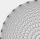Gabo draws n-gon, which angles are consecutive members of an arithmetic sequence. The smallest angle is 70° biggest 170°. How many sides have Gabo's n-gon?
• Polygon 42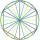Which polygon has 42 more diagonals than sides?
• AnglesThe triangle is one outer angle 158°54' and one internal angle 148°. Calculate the other internal angles of a triangle.
• Internal and external anglesCalculate the remaining internal and external angles of a triangle, if you know the internal angle γ (gamma) = 34 degrees and one external angle is 78 degrees and 40 '. Determine what kind of triangle it is from the size of its angles.
• GlassHow many glass are needed to produce glass with base regular 5-gon if one base triangle in the base is 4.2 square cm and the height is 10 cm?
• One of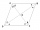One of the internal angles of the rhombus is 120° and the shorter diagonal is 3.4 meters long. Find the perimeter of the rhombus.
• Internal anglesFind the internal angles of the triangle ABC if the angle at the vertex C is twice the angle at the B and the angle at the vertex B is 4 degrees smaller than the angle at vertex A.
• Nine-gonCalculate the perimeter of a regular nonagon (9-gon) inscribed in a circle with a radius 13 cm.
• TowerHow many m2 of the copper plate should be replaced on the roof of the tower conical shape with diameter 24 m, and the angle at the axial section's vertex is 144°?
• N-gon angles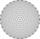What is the sum of interior angles 8-gon? What is the internal angle of a regular convex 8-polygon?
• Alfa, beta, gamaIn the triangle ABC is the size of the internal angle BETA 8 degrees larger than the size of the internal angle ALFA and size of the internal angle GAMA is twice the size of the angle BETA. Determine the size of the interior angles of the triangle ABC.
• Hexagon in circleCalculate the radius of a circle whose length is 10 cm greater than the circumference of a regular hexagon inscribed in this circle.
• Interior angles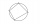In a quadrilateral ABCD, whose vertices lie on some circle, the angle at vertex A is 58 degrees and the angle at vertex B is 134 degrees. Calculate the sizes of the remaining interior angles.
• Octagonal pyramidFind the volume of a regular octagonal pyramid with height v = 100 and the angle of the side edge with the plane of the base is α = 60°.